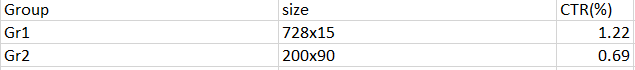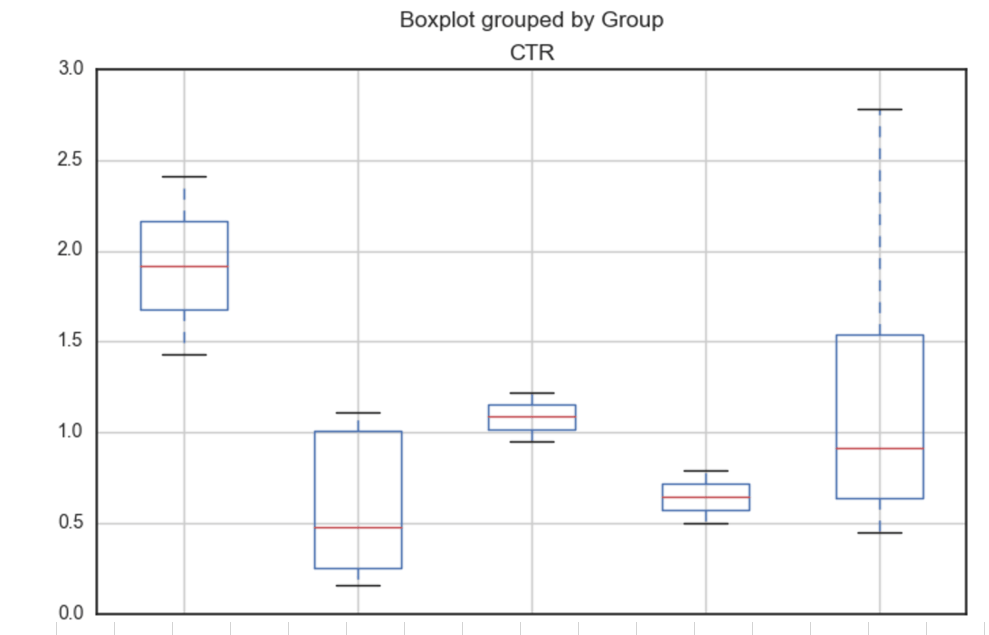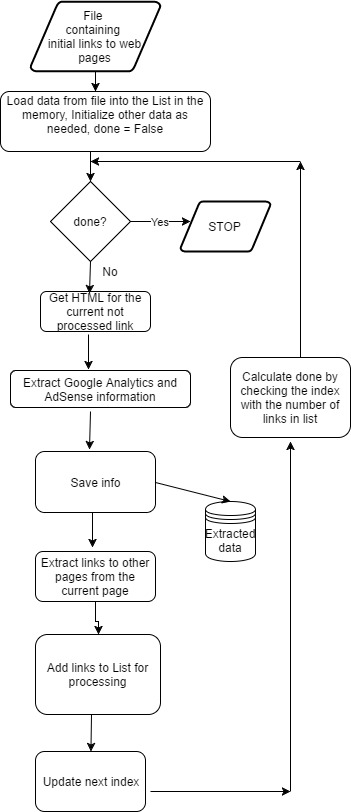## Exploratory Data Analysis (EDA) – No Programming is Needed

In statistics, exploratory data analysis (EDA) is an approach to analyzing data sets to summarize their main characteristics, often with visual methods. A statistical model can be used or not, but primarily EDA is for seeing what the data can tell us beyond the formal modeling or hypothesis testing task. 

According to  Box plot is one of typical graphical techniques used in EDA.

A box plot or boxplot is a convenient way of graphically depicting groups of numerical data through their quartiles. Box plots may also have lines extending vertically from the boxes (whiskers) indicating variability outside the upper and lower quartiles, hence the terms box-and-whisker plot and box-and-whisker diagram. Outliers may be plotted as individual points. 

In this post we will consider how to create box plot for EDA of data from website without using programming. We will use online tool ML Sandbox from this site and the data from Google AdSense and Google Analytics. Here is the sample of few rows:To use the tool we need select “Exploratory Data Analysis” in menu options and then enter data into Input Data Exploratory Data Analysis text field.
Please note that the data should have header field as the first row. Also it is important that the first column should be class column or the column that you use in group by. This field will be on X axis of box plot. It can be text data field. The other columns should be numerical, they will be on Y axis of box plot. In our case we enter data 2 times, with data columns as below:
1. Group, CTR(%) Columns
2. Size, CTR(%) Columns

Each time, after we enter data we click Run Now and then click results link. We might need wait a little and click Refresh button few times untill data results show up.

Here are screenshots of boxplots. We see how the data are distributed for different groups (classes) based on the five number summary: minimum, first quartile, median, third quartile, and maximum.Box plots are useful for identifying outliers and for comparing distributions. Do you want to get the insights into your data? Then visit ML Sandbox and use EDA option to build box plot.

References
1. Exploratory data analysis Wikipedia
2. Box plot Wikipedia
3. ML Sandbox
4. Box Plot: Display of Distribution

Recently I decided to get information that is showing for each page of my website Google Analytics account number and all Google AdSense links on this page. Connecting this information with Google Publisher Pages data would be very useful for better analysis and understanding of ads performance.

So I created python script that is doing the following:

1. Opens file with some initial links. The initial links then are extracted into the list in computer memory.
3. Extracts Google Analytics account number from HTML. The acconneunt number usually appears on web page code on the line, formatted like this : ‘_uacct = “UA-xxxxxxxxxx”;’ The script extracts UA- number using regular expression.
4. Extracts Google AdSense information from HTML text. AdSense information is displayed within /* */ like below:

/* 300×15, created 1/20/17 */
Here ‘300×15, created 1/20/17’ is default ad name.

5. Extracts all links from the same HTML text.
7. Outputs extracted information to csv file. The saved information contains url, GA account number and AdSense ad names that are on the page.
8. Repeats steps 2 – 6 while there are links to process.

Here are few examples how the script can be used:

• Lookup of page for the given ad. For example AdSense is showing clicked link and we want to know what page it was.
• Check if all pages have GA and AdSense code inserted.
• Use the data with Google Analytics and AdSense for analysis of revenue variation or conversion rate by ad size for different group of web pages.

Below you can find script source code and flow chart.

``````
# -*- coding: utf-8 -*-

import urllib.request
import lxml.html
import csv
import time
import os
import re
import string
import requests

path="C:\\Users\\Owner\\Desktop\\2017"

filename = path + "\\" + "urlsB.csv"

filename_info_extracted= path + "\\" + "urls_info_extracted.csv"

urls_to_skip =['search3.cgi']

start=0
file_urls=[]
with open(fn, encoding="utf8" ) as f:
for i, row in enumerate(csv_f):
if i >=  start  :
file_urls.append (row)
return file_urls

def save_extracted_url (fn, row):

if (os.path.isfile(fn)):
m="a"
else:
m="w"

with open(fn, m, encoding="utf8", newline='' ) as csvfile:
fieldnames = ['url', 'GA', 'GS']
writer = csv.DictWriter(csvfile, fieldnames=fieldnames)
if (m=="w"):
writer.writerow(row)

print ("Starting navigate...")

url_ind=0
done=False
while not done:
u=urlsA[url_ind]
new_row={}
print (u)
print (u)

try:
connection = urllib.request.urlopen(u)
print (u)
print ("connected")
time.sleep( 12 )
r = requests.get(u)

# Get the text of the contents
html_content = r.text

pat = re.compile(r"(/\*(.+?)\*/)+", re.MULTILINE)
if pat.search(html_content) != None:

str=""
for match in pat.finditer(html_content):
print ("%s: %s" % (match.start(), match.group(1)))
str=str+","+ match.group(1)
new_row['GS'] = str

pat1 = re.compile(r"_uacct(.+?)\"(.+?)\"", re.MULTILINE)
if pat1.search(html_content) != None:

m=pat1.search(html_content)
new_row['GA'] = m.group(2)

new_row['url'] = u
save_extracted_url (filename_info_extracted, new_row)
url_ind=url_ind+1

print (html_content)

if "lwebzem" in a:

try:

if ind >=0:
else :

except :

skip = False
skip=True
skip = True